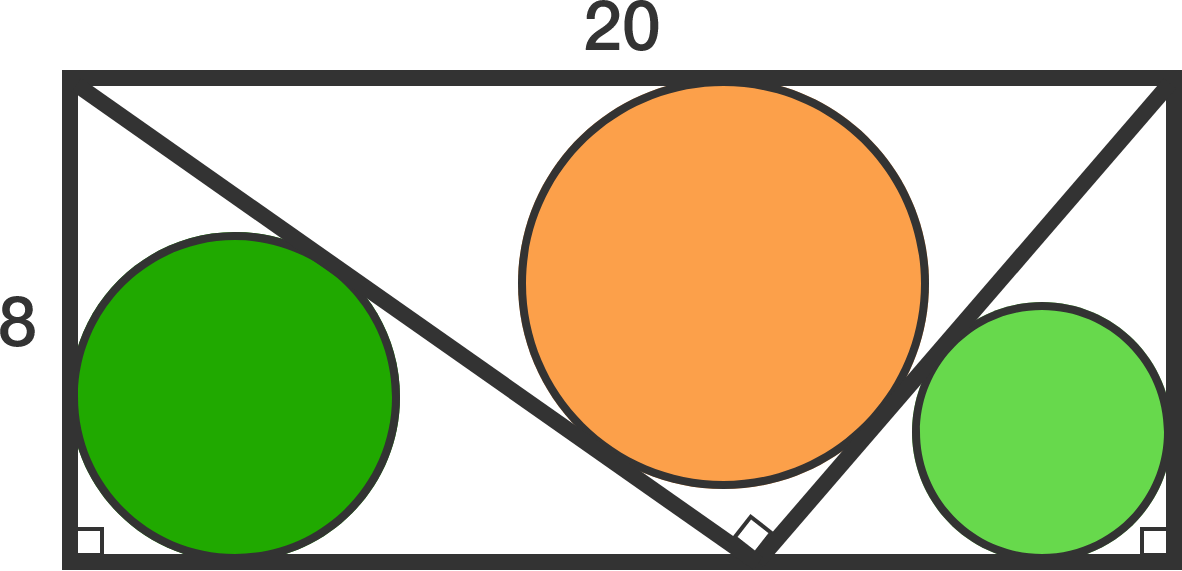# What is the sum of the radii of the three incircles

Geometry Level 2

A $20\times8$ rectangle is partitioned into three right triangles, and a circle is inscribed in each triangle.

Find the sum of the radii of these 3 circles.×